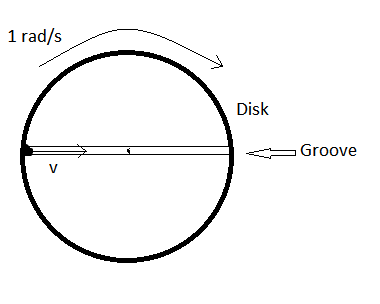# Groovy grooveWe have a disk of mass $M$ and radius $R$ placed on a horizontal plane. A cylindrical groove of radius $r$ is made on a diameter.Now a sphere of mass $m$ and radius $r$ is placed in the groove at the circumference of the disk.

At $t=0$ the whole system is rotated with an angular velocity $1 rad/s$ as shown in the figure.Also at the same time the sphere was projected with a velocity $v$ towards the centre of the disk.Find the minimum velocity $v$ of the sphere such that it is able to reach the other end of the groove.

Details and Assumptions

$1)r\ll R$

$2)M=2kg$

$3)m=3Kg$

$4)R=2 m$

$5)$The disk is fixed about it's centre that is it can only rotate

×

Problem Loading...

Note Loading...

Set Loading...